# Bridge Scour Evauluation

Channel Studio follows the computational methods described in Hydrologic Engineering Circular No. 18, (HEC-18) for scour calculations. After performing the water surface calculations, and if the Scour input for D50 has been entered, scour is automatically calculated. There’s nothing else you need to input.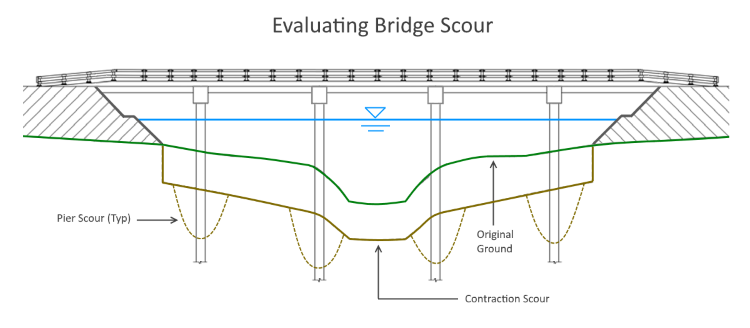The software computes two types of scour:

1. Contraction
2. Pier

Both are described below.

## Contraction Scour

Contraction scour is evaluated using two basic equations:

1. Live-bed
2. Clear-water

Live-bed contraction scour occurs when there is transport of bed material in the upstream approach section into the bridge cross section. With live-bed scour the area of the contracted section (under the bridge) increases until the transport of sediment out of the contracted section equals the sediment transported in.

Clear-water contraction scour occurs when (1) there is no bed material transport from the upstream reach into the downstream reach, or (2) the material being transported in the approach section is transported through the bridge section in suspension.

If the flow in the approach section upstream of the bridge is transporting bed material then it’s live-bed and the Live-bed equation is used. If the flow is not transporting bed material, then it’s clear-water and that equation is used. To determine which condition exists, the critical velocity, the velocity at which bed material begins to transport, must be computed.

### Critical Velocity

Critical Velocity is computed using the following equation: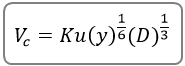Where:

Vc = Critical velocity in ft/s (m/s)
Ku = 11.17 English (6.19 SI)
y = Average depth of flow upstream of the bridge, ft. (m)
D = D50 in ft. (m)

If Vc >= average velocity in the upstream approach section, then the Clear-water equation is used. If not, the Live-bed equation is used. Our experience shows that Live-bed equation produces more scour than the Clear-water equation and there does not appear to be a transition zone. Because of this, you may experience large jumps in scour depths resulting from very small changes in D.

### Live-bed Equation

The Live-bed equation is utilized as needed for each sub-area, i.e., the Left overbank, Main channel and Right overbank.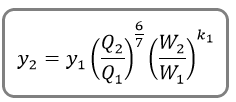Where:

y2 = Average depth in the contracted Bridge section after scour, ft (m)
y1 = Average depth in the upstream approach section, ft (m)
Q1 = Flow in the upstream approach section transporting sediment, cfs (cms)
Q2 = Flow in the bridge section, cfs (cms)
W1 = Bottom width of the approach section that is transporting bed material, ft (m)
W2 = Bottom width of bridge section less pier width(s), ft (m)
k1 = Exponent based on the Fall Velocity of the bed material, D50. Can be 0.59, 0.64 or 0.69

### Clear-water Equation

The Clear-water equation is utilized as needed for each sub-area, i.e., the Left overbank, Main channel and Right overbank.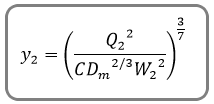Where:

y2 = Average depth in the contracted Bridge section after scour, ft (m)
Q2 = Flow in the bridge section, cfs (cms)
W2 = Bottom width of bridge section less pier width(s), ft (m)
C = 130 for English and 40 for SI units
Dm = 1.25 x D50

### Scour Depth

The actual scour depth, ys, is computed as: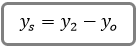Where:

ys = Average depth of scour in ft (m)
yo = Average depth under the bridge (area of flow / top-width) before scour in ft (m)

The bottom widths of sections (W) are approximated by using the computed water surface top widths.

### Pressure Flow Scour

When the water surface at the upstream end of the bridge deck is above the low chord of the deck, pressure flow exists. In these cases a distance, t (separation zone thickness), is added to the computed scour. The distance t is measured from the low chord elevation to the water surface at the downstream end of the deck.

## Pier Scour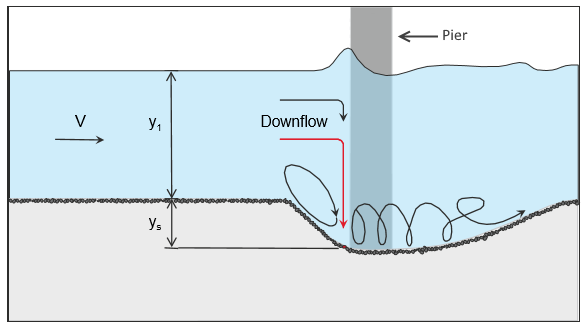Channel Studio uses an equation developed by Dr. David Froehlich, 1991 for computing local pier scour. This equation is used in lieu of the HEC-18 Colorado State University equation due to its simplicity. This equation is also used as an alternate in HEC-RAS.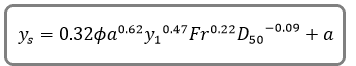Where:

Ø = Correction factor for pier nose shape – 1.0 rounded; 1.3 square; 0.7 triangular
a = Projected pier width with respect to the direction of flow
Fr = Froude number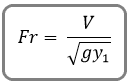Where:

V = Average velocity across the bridge section at the upstream side
y1 = Average depth of flow just upstream of the individual pier

It should be noted that the additional + a (one pier width) at the end of this equation is simply a factor of safety recommended by Froehlich when designing. Channel Studio does not include this in its calculations.

The Pier Scour width is equal to 2 x Ys, to each side of the pier.

### Pier Scour Limits

If the Froude Number, Fr, is <= 0.8 then the maximum pier scour depth is 2.4 x Pier Width. If greater than 0.8 the maximum pier scour width is 3 x Pier Width.

Updated on October 13, 2017